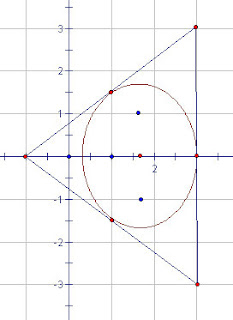## Sunday, 10 January 2010

### Zeros and More Zeros,

An Interesting Relationship Between the Zeros of a Polynomial and the Zeros of Its Derivative.

Just reread an article from Dan Kalman at American University a while back which is here if you want to read the original inspiration for what I am blabbing on about.

Now with real valued polynomials, it is easy to show that between any two zeros of the function, the derivative will have a zero as well, this is essentially what Rolle's theorem says... (Strange that he is remembered most for a "calculus" theorem when he spent much of his life speaking out against calculus). I don't think I knew before I first read this, though, that the average value of the zeros of the polynomial is the same as the average of the zeros of the derivative. Dan's paper shows that this property is true, even if we extend to complex polynomials.

Now I don't spend a lot of time in my high school classes on complex polynomials, but the geometry involved was really nice. It seems that if you plot the zeros of the polynomial (real or complex) on a coordinate plane [complex zero a+bi is plotted as the point (a,b)] and then plot the zeros of the derivative, they will always be inside the boundary determined by the derivatives of the original function (This is called Lucas' Theorem... "For an arbitrary not identically constant polynomial, the zeros of its derivatives lie in the smallest convex polygon containing the zeros of the original polynomial."

For a cubic polynomial for instance, if the roots are not all real, then there will be two complex roots so on the complex plane the three zeros will fall in a triangle. What is really clever about the theorem that Dan has called "Marden's Theorem", is that the two zeros of the derivative of the cubic will not just be inside the triangle formed by the three, but it will be the focus of the ellipse inscribed in the triangle touching the midpoints of the three sides. This means they are symmetric about the centroid of the triangle. The image below shows my rough interpretation of the zeros of x3-7x2+24x-18 which are at 3+3i, 3-3i, and -1. The two zeros of the derivative would then be at 7/3 (+/-) i sqrt(23)/3. And since the second derivative is linear (6x2-14) then the average of the roots will be at x=7/3, which is the centroid of the triangle.Now when the roots are all real, this confirms what most good calculus student's observe pretty quickly, the two zeros of a cubic's derivative are symmetric about the point ofinflection (they have the same average).

SOOOoooooo, he wonders... what happens if we go to a fourth degree polynomial. Time to play.

Ok, so if the polynomial has real coefficients (and if everyone hasn't already dozed off in boredom by now) I just leaned about something called Jensen's Theorem...
which seems to narrow the location of possible zeros of the derivatives a little more. If the polynomial coefficients are real, then all the complex zeros will occur in conjugate pairs (Alg II, you remember!). It seems that if the derivative has any complex zeros, they will have to occur in a circle whose diameter has endpoints on a pair of these conjugate zeros.